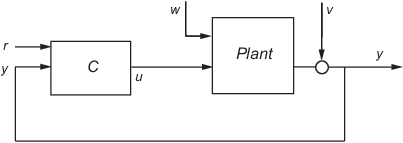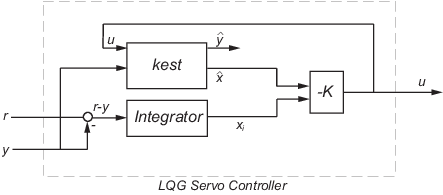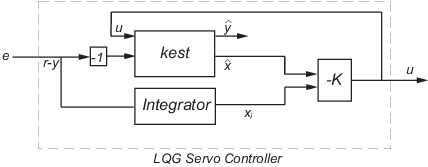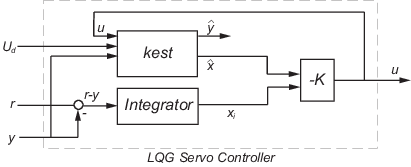# lqgtrack

## Syntax

```C = lqgtrack(kest,k) C = lqgtrack(kest,k,'2dof') C = lqgtrack(kest,k,'1dof') C = lqgtrack(kest,k,...CONTROLS) ```

## Description

`lqgtrack` forms a Linear-Quadratic-Gaussian (LQG) servo controller with integral action for the loop shown in the following figure. This compensator ensures that the output y tracks the reference command r and rejects process disturbances w and measurement noise v. `lqgtrack` assumes that r and y have the same length.Note

Always use positive feedback to connect the LQG servo controller C to the plant output y.

`C = lqgtrack(kest,k)` forms a two-degree-of-freedom LQG servo controller `C` by connecting the Kalman estimator `kest` and the state-feedback gain `k`, as shown in the following figure. `C` has inputs $\left[r;y\right]$ and generates the command $u=-K\left[\stackrel{^}{x};{x}_{i}\right]$, where$\stackrel{^}{x}$ is the Kalman estimate of the plant state, and xi is the integrator output.The size of the gain matrix `k` determines the length of xi. xi, y, and r all have the same length.

The two-degree-of-freedom LQG servo controller state-space equations are

`$\begin{array}{l}\left[\begin{array}{c}\stackrel{˙}{\stackrel{^}{x}}\\ {\stackrel{˙}{x}}_{i}\end{array}\right]=\left[\begin{array}{cc}A-B{K}_{x}-LC+LD{K}_{x}& -B{K}_{i}+LD{K}_{i}\\ 0& 0\end{array}\right]\left[\begin{array}{c}\stackrel{^}{x}\\ {x}_{i}\end{array}\right]+\left[\begin{array}{cc}0& L\\ I& -I\end{array}\right]\left[\begin{array}{c}r\\ y\end{array}\right]\\ u=\left[\begin{array}{cc}-{K}_{x}& -{K}_{i}\end{array}\right]\left[\begin{array}{c}\stackrel{^}{x}\\ {x}_{i}\end{array}\right]\end{array}$`

Note

The syntax `C = lqgtrack(kest,k,'2dof')` is equivalent to `C = lqgtrack(kest,k)`.

`C = lqgtrack(kest,k,'1dof')` forms a one-degree-of-freedom LQG servo controller `C` that takes the tracking error e = ry as input instead of [r ; y], as shown in the following figure.The one-degree-of-freedom LQG servo controller state-space equations are

`$\begin{array}{l}\left[\begin{array}{c}\stackrel{˙}{\stackrel{^}{x}}\\ {\stackrel{˙}{x}}_{i}\end{array}\right]=\left[\begin{array}{cc}A-B{K}_{x}-LC+LD{K}_{x}& -B{K}_{i}+LD{K}_{i}\\ 0& 0\end{array}\right]\left[\begin{array}{c}\stackrel{^}{x}\\ {x}_{i}\end{array}\right]+\left[\begin{array}{c}-L\\ I\end{array}\right]e\\ u=\left[\begin{array}{cc}-{K}_{x}& -{K}_{i}\end{array}\right]\left[\begin{array}{c}\stackrel{^}{x}\\ {x}_{i}\end{array}\right]\end{array}$`

`C = lqgtrack(kest,k,...CONTROLS)` forms an LQG servo controller `C` when the Kalman estimator `kest` has access to additional known (deterministic) commands Ud of the plant. In the index vector `CONTROLS`, specify which inputs of `kest` are the control channels u. The resulting compensator C has inputs

• [Ud ; r ; y] in the two-degree-of-freedom case

• [Ud ; e] in the one-degree-of-freedom case

The corresponding compensator structure for the two-degree-of-freedom cases appears in the following figure.## Examples

See the example Design an LQG Servo Controller.

## Tips

You can use `lqgtrack` for both continuous- and discrete-time systems.

In discrete-time systems, integrators are based on forward Euler (see `lqi` for details). The state estimate $\stackrel{^}{x}$ is either x[n|n] or x[n|n–1], depending on the type of estimator (see `kalman` for details).

For a discrete-time plant with equations:

`$\begin{array}{c}x\left[n+1\right]=Ax\left[n\right]+Bu\left[n\right]+Gw\left[n\right]\\ y\left[n\right]=Cx\left[n\right]+Du\left[n\right]+Hw\left[n\right]+v\left[n\right]\text{ }\left\{\text{Measurements}\right\}\end{array}$`

connecting the "current" Kalman estimator to the LQR gain is optimal only when $E\left(w\left[n\right]v{\left[n\right]}^{\prime }\right)=0$ and y[n] does not depend on w[n] (H = 0). If these conditions are not satisfied, compute the optimal LQG controller using `lqg`.

## Version History

Introduced in R2008b